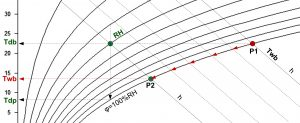# Wet bulb temperature

Wet bulb temperature: Twb [°C]

The air is humidified by evaporating the water until a saturated state is reached. If the evaporated water already has the ambient air temperature, only latent heat is required for its evaporation, which is removed from the air by reducing the sensible heat (evaporative cooling). In this situation, therefore, a state change occurs at constant enthalpy until a saturated state is reached (intersection with the saturation curve, i.e. 100% RH). The temperature of this intersection of the isoenthalpy with the saturation curve is referred to in air conditioning technology as the “wet bulb temperature” or the “adiabatic cooling limit”.

At saturation, the wet bulb temperature is equal to the dry bulb temperature (Twb = Tdb)

Numerical determination of wet bulb temperature Twb is based on the publication presented here.
The iteration starts at RH = 100%, ie Twb = Tdb and proceeds from point P1 to P2.

When writing the code, the input values will be: Tdb [°C], RH [%] and p [atm]:

Twb: = Tdb;
Repeat
Twb: = Twb-0,001;
Pwv: = 611,2 * exp (17 502 * Twb / (240,97 + Twb)) – 66,8745 * (1 + 0,00115 * Twb) * p * (Tdb – Twb);
Pswv: = 6,112 * exp (17 502 * Tdb/ (240,97 + Tdb));
RHs: = Pwv / Pswv;
until (RHs <= RH);
print Twb